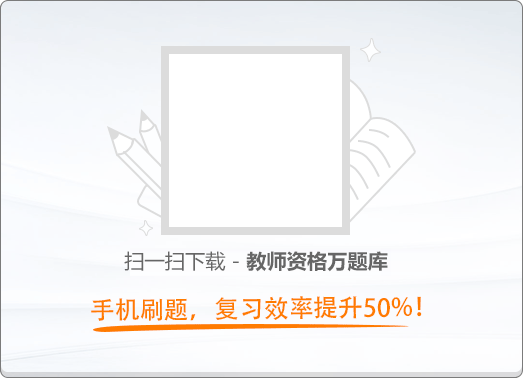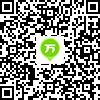120:002019年3月《幼儿保教知识与能力》真题

1
(单项选择题)

• A.

保教幼儿和服务家长

• B.

看护幼儿和服务家长

• C.

培养习惯和态度

• D.

保育和教育幼儿

• A
• B
• C
• D

2
(单项选择题)

• A.

注意的选择性

• B.

注意的广度

• C.

注意的稳定性

• D.

注意的分配

• A
• B
• C
• D

3
(单项选择题)

• A.

具体形象性

• B.

抽象逻辑性

• C.

直观动作性

• D.

不可逆性

• A
• B
• C
• D

4
(单项选择题)

• A.

情感目标

• B.

认知目标

• C.

动作技能目标

• D.

行为目标

• A
• B
• C
• D

5
(单项选择题)

• A.

情感表达

• B.

自我反思

• C.

自我调节

• D.

交流信息

• A
• B
• C
• D

6
(单项选择题)

• A.

淋巴系统

• B.

生殖系统

• C.

神经系统

• D.

消化系统

• A
• B
• C
• D

7
(单项选择题)

• A.

阶段性原则

• B.

社会原则

• C.

操作性原则

• D.

差异性原则

• A
• B
• C
• D

8
(单项选择题)

• A.

幼儿学习活动的指导者

• B.

幼儿学习活动的管理者

• C.

幼儿学习活动的设计者

• D.

幼儿学习活动的合作者

• A
• B
• C
• D

9
(单项选择题)

• A.

支持幼儿表达自己对美术作品的独特感受

• B.

出示范画让幼儿模仿

• C.

鼓励幼儿用自己的方式表现美

• D.

为幼儿的美术创作提供丰富的材料

• A
• B
• C
• D

10
(单项选择题)

• A.

认识和命名更多的几何图形

• B.

默数，接着数等计数能力

• C.

以一一对应的方式数10个以内的物体。并说出总数

• D.

通过实物操作进行10以内加、减法的运算能力

• A
• B
• C
• D